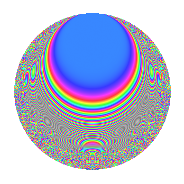# Properties

 Label 40.6.kLevel 40 Weight 6 Character orbit k Rep. character $$\chi_{40}(3,\cdot)$$ Character field $$\Q(\zeta_{4})$$ Dimension 56 Newform subspaces 1 Sturm bound 36 Trace bound 0

# Related objects

## Defining parameters

 Level: $$N$$ $$=$$ $$40 = 2^{3} \cdot 5$$ Weight: $$k$$ $$=$$ $$6$$ Character orbit: $$[\chi]$$ $$=$$ 40.k (of order $$4$$ and degree $$2$$) Character conductor: $$\operatorname{cond}(\chi)$$ $$=$$ $$40$$ Character field: $$\Q(i)$$ Newform subspaces: $$1$$ Sturm bound: $$36$$ Trace bound: $$0$$

## Dimensions

The following table gives the dimensions of various subspaces of $$M_{6}(40, [\chi])$$.

Total New Old
Modular forms 64 64 0
Cusp forms 56 56 0
Eisenstein series 8 8 0

## Trace form

 $$56q - 2q^{2} - 4q^{3} + 176q^{6} + 244q^{8} + O(q^{10})$$ $$56q - 2q^{2} - 4q^{3} + 176q^{6} + 244q^{8} - 570q^{10} - 8q^{11} - 308q^{12} + 456q^{16} - 408q^{17} + 3370q^{18} + 1360q^{20} - 3148q^{22} - 3120q^{25} - 5084q^{26} + 968q^{27} - 10020q^{28} + 21100q^{30} + 7968q^{32} - 976q^{33} - 4780q^{35} + 23132q^{36} - 49524q^{38} - 58980q^{40} - 8q^{41} + 42060q^{42} + 1308q^{43} + 42416q^{46} + 93776q^{48} - 94010q^{50} + 20872q^{51} - 67180q^{52} - 43384q^{56} + 968q^{57} + 86100q^{58} + 118180q^{60} + 6240q^{62} + 17680q^{65} - 41128q^{66} + 89252q^{67} - 158164q^{68} + 116980q^{70} + 1180q^{72} - 25184q^{73} - 127740q^{75} + 41592q^{76} - 161440q^{78} - 167360q^{80} - 67792q^{81} + 23412q^{82} - 126444q^{83} - 192704q^{86} + 100176q^{88} - 82330q^{90} + 329432q^{91} + 226020q^{92} + 358176q^{96} + 212576q^{97} + 65834q^{98} + O(q^{100})$$

## Decomposition of $$S_{6}^{\mathrm{new}}(40, [\chi])$$ into newform subspaces

Label Dim. $$A$$ Field CM Traces $q$-expansion
$$a_2$$ $$a_3$$ $$a_5$$ $$a_7$$
40.6.k.a $$56$$ $$6.415$$ None $$-2$$ $$-4$$ $$0$$ $$0$$

## Hecke characteristic polynomials

There are no characteristic polynomials of Hecke operators in the database